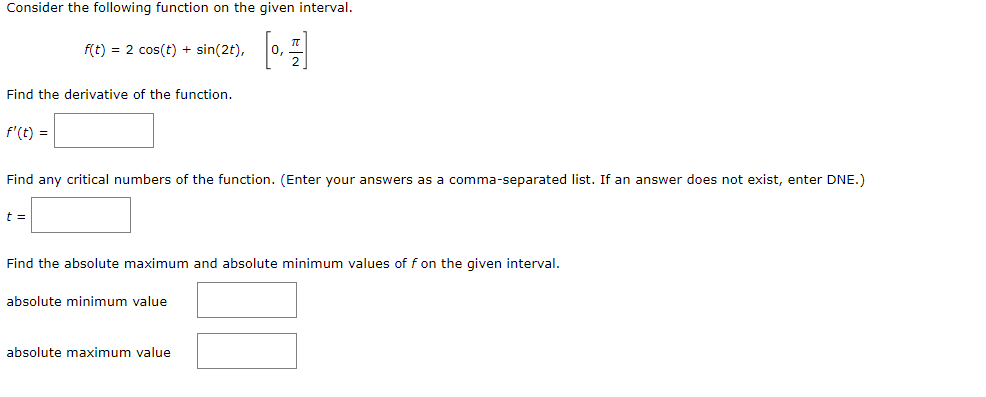Home / Expert Answers / Calculus / consider-the-following-function-on-the-given-interval-f-t-2cos-t-sin-2t-0-2-find-the-der-pa591

# (Solved): Consider the following function on the given interval. f(t)=2cos(t)+sin(2t),[0,2] Find the der ...Consider the following function on the given interval. Find the derivative of the function. Find any critical numbers of the function. (Enter your answers as a comma-separated list. If an answer does not exist, enter DNE.) Find the absolute maximum and absolute minimum values of on the given interval. absolute minimum value absolute maximum value

We have an Answer from Expert